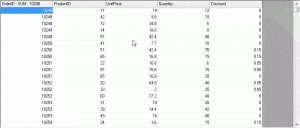C1FlexGrid is a grid control for creating user-friendly interfaces that display, edit, format, organize, summarize, and print tabular data. This blog deals with yet another utility implementation of C1FlexGrid for Winforms. It guides the user on how to calculate the sum of the selected cells in a column & display the same in the respective column's header using the Grid's SelChange & AfterSelChange events.

## Calculate the Sum

In the SelChange event of C1FlexGrid, we will retrieve the respective column's Caption and Index & will loop through the number of selected cells in this column. Thereby, we will calculate the sum of the contents in this selection.

``````

private void c1FlexGrid1_SelChange(object sender, EventArgs e)
{
total = null;

//Get the respective Column's Caption & Index
if (col_index == -1)
col_name = c1FlexGrid1.Cols[c1FlexGrid1.Col].Caption;

else if (c1FlexGrid1.Col != col_index)
{
c1FlexGrid1.Cols[col\_index].Caption = col\_name;
col_name = c1FlexGrid1.Cols[c1FlexGrid1.Col].Caption;
}
col_index = c1FlexGrid1.Col;

double sum\_of\_cells = 0;

//Calculate the Sum of the Selection
if (c1FlexGrid1.Cols[c1FlexGrid1.Col].DataType != System.Type.GetType("System.String"))
{
for (int i = c1FlexGrid1.Row; i <= c1FlexGrid1.RowSel; i++)
sum\_of\_cells = sum\_of\_cells + Convert.ToDouble(c1FlexGrid1[i, c1FlexGrid1.Col]);
}
else
MessageBox.Show("String Column!");
total = col\_name + " - SUM : " + sum\_of_cells;
}

``````

## Display the Sum

After calculating the sum, we will display the sum in the respective Column's Header using the AfterSelChange event of the Grid. The resultant output will be displayed as the Column's Caption.

``````

private void c1FlexGrid1_AfterSelChange(object sender, C1.Win.C1FlexGrid.RangeEventArgs e)
{
//Display the Sum in column Header
c1FlexGrid1.Cols[c1FlexGrid1.Col].Caption = total;
}

``````

Click the image below for a better understanding of the behavior.Please refer to the attached samples for complete implementation. Download Sample CS Download Sample VB